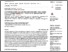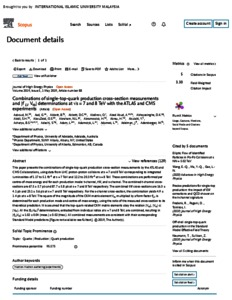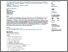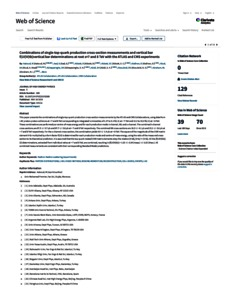# Combinations of single-top-quark production cross-section measurements and |f LV Vtb| determinations at √s = 7 and 8 TeV with the ATLAS and CMS experiments

Aaboud, M. and Aad, Georges and Abbott, Brad K. and Abbott, D. C. and Abdinov, O. B. and Md. Ali, Mohd. Adli (2019) Combinations of single-top-quark production cross-section measurements and |f LV Vtb| determinations at √s = 7 and 8 TeV with the ATLAS and CMS experiments. Journal of High Energy Physics, 2019 (5). pp. 1-80. ISSN 1029-8479PDF - Published Version
Restricted to Repository staff onlyPreview
PDF (Scopus) - Supplemental MaterialPreview
PDF (wos) - Supplemental Material

## Abstract

This paper presents the combinations of single-top-quark production crosssection measurements by the ATLAS and CMS Collaborations, using data from LHC proton–proton collisions at √ s = 7 and 8 TeV corresponding to integrated luminosities of 1.17 to 5.1 fb−1 at √ s = 7 TeV and 12.2 to 20.3 fb−1 at √ s = 8 TeV. These combinations are performed per centre-of-mass energy and for each production mode: tchannel, tW, and s-channel. The combined t-channel cross-sections are 67.5 ± 5.7 pb and 87.7 ± 5.8 pb at √ s = 7 and 8 TeV respectively. The combined tW cross-sections are 16.3 ± 4.1 pb and 23.1 ± 3.6 pb at √ s = 7 and 8 TeV respectively. For the schannel cross-section, the combination yields 4.9 ± 1.4 pb at √ s = 8 TeV. The square of the magnitude of the CKM matrix element Vtb multiplied by a form factor fLV is determined for each production mode and centre-of-mass energy, using the ratio of the measured cross-section to its theoretical prediction. It is assumed that the top-quark-related CKM matrix elements obey the relation |Vtd|, |Vts| � |Vtb|. All the |fLVVtb| 2 determinations, extracted from individual ratios at √ s = 7 and 8 TeV, are combined, resulting in |fLVVtb| = 1.02 ± 0.04 (meas.) ± 0.02 (theo.). All combined measurements are consistent with their corresponding Standard Model predictions.

Item Type: Article (Journal) 7861/79576 Hadron-Hadron scattering (experiments) Q Science > Q Science (General)Q Science > QC Physics Kulliyyah of Science > Department of Physics Dr Mohd Adli MD Ali 16 Mar 2020 15:53 16 Mar 2020 15:53 http://irep.iium.edu.my/id/eprint/79576View Item